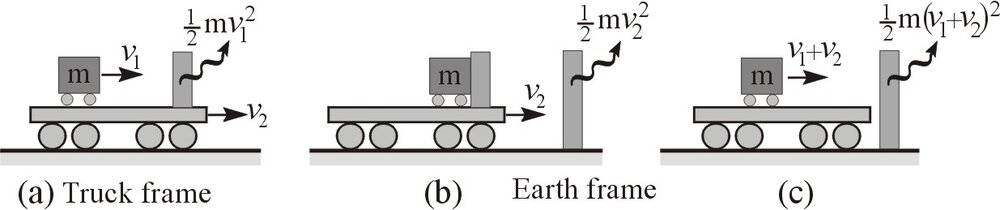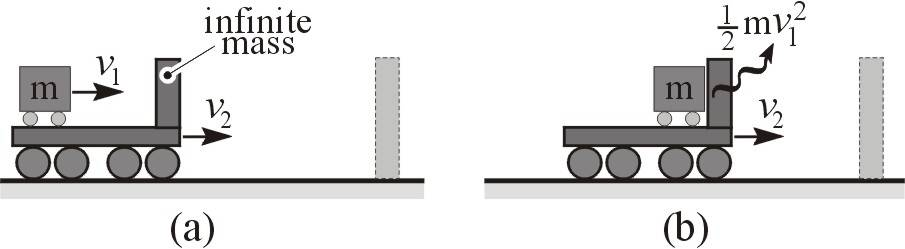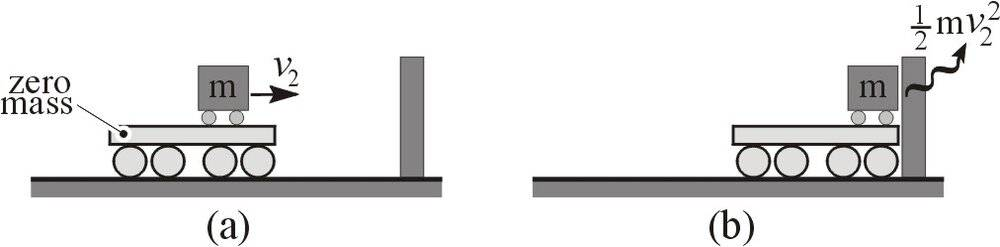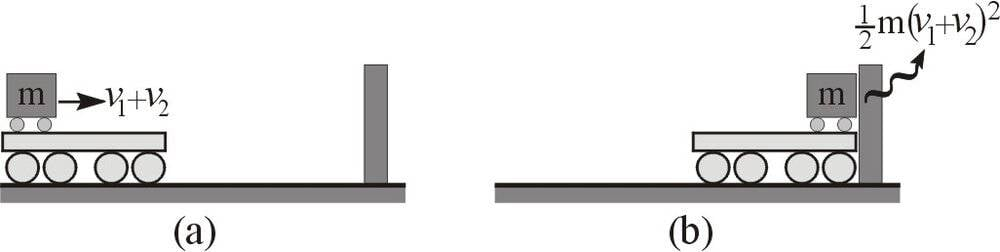# Kinetic energies in two reference frames

jeremyfiennes
A very basic and simple query, but I can't see my way through it.A mass m moves at speed v1 relative to a truck traveling at speed v2 , fig.a. All components except this mass are massless.
In a truck-stationary frame, the mass collides with a barrier on the truck liberating kinetic energy 1/2.mv1^2.
Switching to an Earth-stationary frame, the mass+truck continue at v2 and collide with an Earth-fixed barrier liberating a further kinetic energy 1/2.mv2^2, fig.b.
Had, however, the mass from the start gone straight on to collide with the Earth-fixed barrier, fig.c, it would have liberated kinetic energy 1/2.m(v1+v2)^2.
How can energy apparently vanish on switching frames?

Mentor
Your scenario is impossible. Momentum is not conserved. You need to redo your scenario to conserve momentum and then energy will work out in both frames.

•nasu, Vanadium 50 and Doc Al
jeremyfiennes
Not with you. How?

Mentor
A single massive object cannot stop if everything else is massless. The massless objects cannot conserve momentum, regardless of how fast they move after the collision.

Once you have massive objects to collide with, the conservation of energy and momentum both work in all frames.

Last edited:
Your OP is unfortunately non-sense. Consider a cart of mass ##M## initially moving at ##u_c## relative to the Earth, on which a block of mass ##m## slides initially at ##u_b## relative to the cart. We will assume any collisions are perfectly inelastic. Let's just analyse a single collision, to iron out the Physics.

There is a rigid barrier attached to the front of the cart, with which the sliding block eventually collides and coalesces. After this collision, the velocity of the (cart + block) system relative to the Earth is given by
$$V = \frac{Mu_c + m(u_b + u_c)}{m + M}$$It's easy to verify that the decrease in kinetic energy after this collision is\begin{align*}

-\delta E &= \frac{1}{2}m(u_c + u_b)^2 + \frac{1}{2} Mu_c^2 - \frac{1}{2}(m+M) \left( \frac{Mu_c + m(u_b + u_c)}{m + M} \right)^2 \\

&= \frac{1}{2}m(u_c + u_b)^2 + \frac{1}{2} Mu_c^2 - \frac{M^2 u_c^2 }{2(m+M)} - \frac{mMu_c(u_b + u_c)}{m+M} - \frac{m^2(u_b + u_c)^2}{2(m+M)} \\

&= \frac{1}{2} \frac{mM}{m+M} \left[ (u_c + u_b)^2 + u_c^2 - 2u_c (u_c + u_b)\right] \\

&= \frac{1}{2} \frac{mMu_b^2}{m+M}

\end{align*}Meanwhile in the centre-of-mass frame of the system, the initial velocities (this time both relative to the centre-of-mass) are\begin{align*}

\end{align*}thus the kinetic energy differential in the centre-of-mass frame (in which the two bodies come completely to rest after the inelastic collision) is\begin{align*}

-\delta E' = \frac{1}{2}m \frac{M^2u_b^2}{(m+M)^2} + \frac{1}{2} M \frac{m^2u_b^2}{(m+M)^2} = \frac{1}{2} \frac{mM u_b^2}{m+M}
\end{align*}and you'll notice that ##\delta E = \delta E'##, which reflects the frame invariant fact that this is the amount of energy dissipated as heat, etc.

You'll notice I didn't bother with the truck-fixed frame here. It's not inertial, so there is not an energy conservation law in the usual sense. As the sliding block comes to rest in a short time ##\delta t## in the truck-fixed frame, it's acted upon by an inertial force ##-m\mathbf{A}## whose work through that tiny displacement needs to be accounted for in order to recover energy conservation.

Last edited by a moderator:
•Richard R Richard and Dale
Homework Helper
Summary:: Kinetic energies in two reference frames: where does the difference go?

A very basic and simple query, but I can't see my way through it.

View attachment 281858

A mass m moves at speed v1 relative to a truck traveling at speed v2 , fig.a. All components except this mass are massless.
In a truck-stationary frame, the mass collides with a barrier on the truck liberating kinetic energy 1/2.mv1^2.
Switching to an Earth-stationary frame, the mass+truck continue at v2 and collide with an Earth-fixed barrier liberating a further kinetic energy 1/2.mv2^2, fig.b.
Had, however, the mass from the start gone straight on to collide with the Earth-fixed barrier, fig.c, it would have liberated kinetic energy 1/2.m(v1+v2)^2.
How can energy apparently vanish on switching frames?
To state it explicitly: The Earth gains (or loses) a tiny bit of velocity in the collision.

In the frame where the Earth is at rest before the collision, the resulting kinetic energy gain by the Earth scales with the square of that tiny bit of velocity change -- negligible, even though it also scales with the mass of the Earth. (##\Delta KE_\text{earth} = \frac{1}{2}m(\Delta v_\text{earth})^2##)

##m## = mass of Earth, ##V## = velocity of truck in Earth frame, ##\Delta v## = change in velocity of the Earth as a result of the collision.

In the frame where the Earth approaching at the speed of a truck before the collision, the resulting kinetic energy loss by the Earth scales linearly with the velocity change -- non-negligible because it also scales with the mass of the Earth. (##\Delta KE_\text{earth} = \frac{1}{2}mV^2 - \frac{1}{2}m(V-\Delta v_\text{earth})^2## which, if you work it out is larger than the figure above by exactly ##mV\Delta v##).

Note that ##m \Delta v## is equal to the momentum of the moving truck as measured in the Earth frame. Multiply by V and you have something non-negligible.

jeremyfiennes
Thanks all. This has however complicated it beyond my original intention, which was the KE of the small mass m only in the two frames. Without bringing in the rest of the system. Maybe it can be separated into 3 parts.
- 1) the truck has infinite mass. Ek = 1/2.mv1^2 is liberated without changing the truck speed..-2) this truck is instantaneously substituted by one with zero mass and no barrier. The position and velocity of the small mass m remain unchanged. On collison with the end barrier, Ek = 1/2.mv2^2 is liberated. Agreed that the Earth will move a fraction, but this will not significantly affect v2.- 3) all in one. The small mass goes from the beginning to the end with a zero mass truck. Ek = 1/2.m(v1+v2)^2 is liberated.So Ek = 2.v1.v2 has gone missing somewhere.

jeremyfiennes
Sorry, Ek = m.v1.v2 missing.

...the truck has infinite mass... this truck is instantaneously substituted by one with zero mass ... Ek = 2.v1.v2 has gone missing somewhere.
Your scenario doesn't even have mass conservation but you expect energy to be conserved?

•etotheipi
Mentor
So Ek = 2.v1.v2 has gone missing somewhere.
The missing energy is down at the local pub sharing a pitcher of beer with the momentum that also snuck out during the collision with a barrier of infinite mass.

OK, seriously, kidding aside... Neither energy nor momentum is conserved in collisions involving infinite masses, and that’s what you’re seeing here. This would be a major problem for physics if there were such a thing as an infinite mass, but of course there is not - in fact, your thought experiment can be understood as a proof that an infinite mass is physically impossible.

You might reasonably ask why we would introduce an infinite mass into any problem then. The answer is that it simplifies the calculation and can still be a very good approximation. An exact solution for the collision with the barrier will involve energy and momentum terms proportional to ##m/M_b##, where ##M_b## is the mass of the barrier; when we say the barrier has infinite mass we’re really saying that we will ignore those terms. Ignoring them unsurprisingly leads to some “missing” energy and momentum.

(You gave yourself a big hint in your post #7 when you said that the Earth’s velocity does not change “significantly”... even an insignificant change in the earth’s speed involves amounts of energy and momentum that are significant to us).

••nasu and Dale
Mentor
This has however complicated it beyond my original intention, which was the KE of the small mass m only in the two frames. Without bringing in the rest of the system.
If you don't bring in the rest of the system then you cannot be surprised that energy is not conserved.
the truck has infinite mass. Ek = 1/2.mv1^2 is liberated without changing the truck speed
If you want to do that correctly then you must start with a finite mass truck, solve the equations, and then take the limit as the mass of the truck goes to infinity. That is the mathematically correct way to do it.

Look, you are trying to take shortcuts and you are winding up with contradictions. The contradictions are a direct result of your shortcuts. If you work out the problem correctly then energy and momentum will both work out in all frames.

If you take shortcuts that neglect important places for energy and momentum to go then you cannot be surprised if they go to those places that you neglected. There is nothing wrong with taking the shortcuts, but there is something wrong with taking the shortcuts if you are interested in exactly the quantities that the shortcuts neglect.

jeremyfiennes
Ok. Thanks. I sit corrected.

Homework Helper
2022 Award
This should not be hard to see because:
1. The KE of any single moving object depends upon frame
2. KE is not conserved (i.e. it goes to un-included degrees of freedom) in any inelastic collision
Why are you surprised?

DrStupid
Maybe it can be separated into 3 parts.
[...]
So Ek = 2.v1.v2 has gone missing somewhere.
No, nothing is missing. You just have different cases with different results.

If a mass ##m## with initial velocity ##v_0## hits a wall with mass ##m_w## and initial velocity ##v_w## in a total inelastic collition they will continue to move with the common velocity

##v = \frac{{m \cdot v_0 + m_w \cdot v_w }}{{m + m_w }}##

(due to conservation of momentum) and the total kinetical energy is reduced by

##\Delta E_{kin} = {\textstyle{1 \over 2}}\left( {m + m_w } \right) \cdot v^2 - {\textstyle{1 \over 2}}m \cdot v_0^2 - {\textstyle{1 \over 2}}m_w \cdot v_w^2 = - {\textstyle{1 \over 2}}\frac{{m \cdot m_w }}{{m + m_w }} \cdot \left( {v_0 - v_w } \right)^2##

As the released energy depends on the relative velocity between mass and wall, it is frame-independent.

If the wall is considered to have infinite masse, the equation is simplified to

##\mathop {\lim }\limits_{m_w \to \infty } \Delta E_{kin} = - {\textstyle{1 \over 2}}m \cdot \left( {v_0 - v_w } \right)^2##

Now you just need to insert the values for the three different cases above:

1) ##v_0 = v_1 + v_2## and ##v_w = v_2## (or ##v_0 = v_1## and ##v_w = 0## in the restframe of the truck)
2) ##v_0 = v_2## and ##v_w = 0##
3) ##v_0 = v_1 + v_2## and ##v_w = 0##